# Deutsch-Jozsa Algorithm

Before we try to understand this quantum algorithm, let’s understand what is the general purpose of a computer to see the significance of this algorithm.

A computer is a physical device that helps us process information by executing algorithms. An algorithm is a set of instructions given to a computer in order to solve a problem.

Algorithms are measured based on the number of resources that are used by a computer in order to solve a problem. Resources can be defined in two ways: time and space.

Time: how long it takes to perform the computation.

Space: Amount of memory used by the computer in performing the computation.

For certain problems, quantum computers are able to clearly outperform classical computers. What makes this algorithm so significant is that it is one of the first concrete representations of the power of a quantum vs a classical computer.

## Important Concepts to Understand

1. Computational basis states

Classical computers have bits of information, the state of a bit is a number (0 or 1). On the other hand, the state of a qubit is a vector, more specifically, the state of a qubit is a vector in a two-dimensional vector space. This two-level system allows for what is called a quantum superposition, to be brief it is the ability to be two states at the same time, for a deeper explanation click here. Below is the representation of the 0 and 1 as vectors.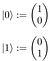Vector Space of ∣0⟩ and∣1⟩

You might be wondering why 0 is written as ∣0⟩ or why 1 written ∣1⟩. These special states are called computational basis states.

It’s tempting to see the ∣0⟩ notation and wonder what all the separate pieces mean — what, for instance, does the |mean; what does the ⟩ mean; and so on?

In fact, it’s best to regard ∣0⟩ as a single symbol, standing for a single mathematical object — that vector we just saw above. The | and ⟩ don’t really have separate meanings except to signify this is a quantum state.

2. Normalization

In quantum systems, in order to create the computation, we need to manipulate their quantum state in a controlled fashion which is difficult to do since qubits are so unstable.

An important axiom (or principal) is that all qubit states are normalized, this means that the state of the system is kept at length 1. When you preserve length 1 throughout you are making sure no information is lost in the computation. When you lose information, your system falls apart due to the reliance of qubits on each other through quantum entanglement.

Normalization through the sum of squares of the amplitudes. For example, for the state 0.6∣0⟩ + 0.8∣1⟩, the amplitudes (coefficients) are the number that are outside the computational basis states. The sum of the squares of the amplitudes is 0.⁶²+0.⁸², which is 0.36+0.64 = 1, as we desired.

Speaking more generally, the amplitudes can be complex numbers, which are conventionally denoted by α and β so the state is α∣0⟩+β∣1⟩. The constraint is now that the sum of the squares of the amplitudes is 1, i.e. ∣α∣²+∣β∣²=1.

3. Unitary Operators

It turns out that unitary operators represented by matrices preserve the length of their inputs. If we go back to the importance of normalization so we don’t lose our quantum information, unitary operators help us get there. In the coming algorithm, it is an important idea to helps us solve this circuit.

4. Reversibility

Reversibility is when based on the output of a gate, we can determine what the inputs are. When we are in a position where we can not reverse output it means that we lost information making our quantum computer or quantum system not work in the desired manner. Our qubits becoming scrambled and unprotected, rendering this process an error.

Below is the example of a classical NOT gate that simply reverses our input.

This circuit is reversible because when we look at our output 0 we will get 1 and if our output is 1 then our input is 0.

If you look below we have the AND gate which is not reversible.

If we have the output 1 then we know that A and B have to be 1. The problem here is we have the output 0 then it could be any of the 3 combinations for the example of a 2-bit input. This lack of info makes it very difficult to properly manipulate our qubits if don’t know what combination we have.

## Background of the Deutsch-Jozsa Algorithm

The Deutsch-Jozsa algorithm was the first example of a quantum algorithm that performs better than the best classical algorithm. It uses quantum properties such as quantum interference (as shown here in the double-slit experiment) that allow us to figure out the solution to our problem.

## What Is The Problem?

We are given a reversible circuit implementing an unknown function f. We are given the promise that our function is either balanced or constant. A constant function returns all 0’s or all 1’s for any input, while a balanced function returns 0’s for exactly half of all inputs and 1’s for the other half. Our task is to determine whether the given function is balanced or constant by making queries to the circuit for f.

We treat this reversible circuit as a ‘black box’ or ‘oracle’ (looks like a black hole in the middle of the circuit). This means that we can apply the circuit to obtain values of f(x) for given inputs x, but we cannot gain any information about the inner workings of the circuit to learn about the function f. In this situation, we have to make queries (or calls) to the function in order to figure out whether f is balanced or constant.

## Classical Solution

In our unknown function f takes n-bit strings as an input. In computer programming, a string is a finite sequence of symbols. In our case, the Deutsch-Jozsa problem binary digits (0 or 1) as values and it returns either 0 or 1. An abstract variable terms a string of bits for the function f looks like this:({x₀, x₁, x ₂,…})→0 or 1, where xₙ is 0 or 1. In more concrete terms, a string of bits for function f may look like this: f(1,0,0,…)→1.

Just to clarify the → is referring to the return value of the string.

Since we work with 2 values 0 and 1 we use the base 2 when we calculate our possibilities. In this situation, in order for us to find the solution we first need to identify how many possibilities that we have. 2ⁿ is how we find the number of combinations based on our n-bit input. For example, let’s say that n=2

²²=4 different combinations of 2-bit strings

Combination 1: 00

Combination 2: 01

Combination 3: 10

Combination 4: 11

Based on any set of combinations, in the best-case scenario, we only need to make two queries. If we get both f(0,0,0,…)→0 and f(1,0,0,…)→1, then we know the function is balanced as we have obtained the two different outputs.

In the worst-case scenario, since 2ⁿ is the total number of possibilities, in order to prove we are 100% certain of whether the function is balanced or constant we need to check half of the possibilities + 1.

Let’s use the example of the 2³, which is a 3-bit string with 8 different possible combinations. If we check 4 out of 8 combinations and we get a return value of 0 every time, even though it is probabilistically unlikely the 5ᵗʰ input returns a 1. If it returns a 1 then we no longer have a constant function, we now have a balanced one. The implication of this is we need to query more than half of our combinations to be 100. To verify a function for any number n is balanced, we query f for

To give a little bit of a background on why this model makes sense. Since 2ⁿ is the total number of possibilities. Our base (2) is what we multiply by every time. If we do 2ⁿ-¹ then we divide our total number by 2 granting us half of the possibilities. The +1 is there to get us passed the half way point to be certain.

## Quantum Solution

With quantum computing, on the other hand, we can limit that query to just 1 and we can be 100% certain of whether function f is constant or balanced. To explain this model we need to examine the circuit and work through the math.

To understand this quantum circuit we first need to be familiar with the Hadamard gate. Below is the value of a Hadamard gate being applied to our two states.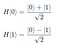Hadamard being applied to ∣0⟩ and ∣1⟩

Now that we have some fundamentals we can get into into the circuit of the algorithm.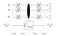The circuit for the Deutsch–Jozsa algorithm

Note that the last input bit has been initialized to the state to the Hadamard ∣1⟩. This gate is not shown for arbitrary reasons, the algorithm will not be any different if you had ∣1⟩ and had Hadamard gates to parallel the ones in the for the circuit lines of ∣0⟩.

At the bottom of the circuit, you will see|ψ₀⟩ to |ψ3⟩. These are different points within the circuit, these are a convenient way to analyze the behavior of this quantum algorithm. This helps us work through the state at each stage of the circuit.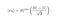First Step in the Circuit

In our first state, we have ∣0⟩⊗n, this means that we using the tensor product of the ∣0⟩ and our n value. In this situation, our tensor products refer to the number of ∣0⟩’s that are involved in our circuit. For example if n=3 then we have 3 one-qubit ∣0⟩ states. The state on the right side of ∣0⟩⊗n is the∣1⟩ state after a Hadamard gate is applied to it.

In this position, we are evaluating the in between the step of |ψ₀⟩ and |ψ₁⟩ where we apply the Hadamard gates. Note, this step does not include∣1⟩ and its transformation with the Hadamard gate as it has already been transformed.

Here we are applying our tensor products to get how many 1-bit input blocks of the Hadamard and ∣0⟩ we need for our circuit. Going back to the example of n=3. We will have 3 Hadamard blocks and 3 ∣0⟩ inputs.

Since the Hadamard gate is unitary (it preserves the lengths of the inputs), it is expected to follow the normalization. To calculate the probability of whether we will get 0 or 1 as a value, we need to square our amplitudes and add them. For a single Hadamard ∣0⟩, we would get ∣0⟩ + ∣1⟩ ÷ √2. If you factor it out you get (1/√2) (∣0⟩ + ∣1⟩). This is the logic behind the line above. The exponent comes from us having more than 1 Hadamard of∣0⟩. With our coefficient of 1/√2, we achieve an equally weighted superposition where there is an equal chance of getting the values ∣1⟩ and ∣0⟩.

In this situation, our starting point (left of the equal sign) is the same as the line before. Our amplitude (or coefficient) is the same. The only difference is that we use Sigma notation which simply says the same thing as the previous line, except it, is a much efficient way of writing it.

It says that x (an n-bit string) is in the elements (consisting) of the values 0 and 1 with the n (outside the curly brackets) dictating how many values are in our string.

To remind you of where we are we just went through the line of H-blocks (Hadamard Gates) on the left side and we have reached|ψ₁⟩. The only difference between this line and the last one is the multiplication of Hadamard ∣1⟩ with the rest of our circuit.

When approaching the unitary function f (Uf) which is also known as a controlled unitary function. In controlled functions, you have the control qubit (the dot) and the target qubit (⊕).

An example of such a gate is the controlled-NOT (or CNOT) gate. In the quantum circuit language, we have two wires, representing two qubits, and the following notation to represent the CNOT gate: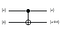Controlled-NOT Gate

Usually, the control qubits control the target qubits by changing their states. If the control qubit is set to ∣1⟩, as in the states ∣10⟩ and ∣11⟩, it flips the target qubit then it flips (i.e., NOTs) the target qubit. And otherwise, it does nothing. Writing out the action on all four computational basis states we have:

∣00⟩→​​∣00⟩

∣01⟩→∣01⟩

∣10⟩→∣11⟩

∣11⟩→∣10⟩​

In this algorithm, the Hadamard states change the roles of the qubits. The control and target qubits are effectively switched where the target qubit affects the control qubit.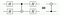How controlled Hadamard changes control gates

This change causes our coefficient to become a -1. Looking below the difference between the Hadamard of ∣0⟩ and ∣1⟩​​, one has a positive basis state, while the other is in a negative basis state.

Using the reversed version of the controlled gate due to our Hadamard states. The control qubit (now the Hadamard of ∣1⟩) affects our target qubits (all of the Hadamards of ∣0⟩).​ ​This causes our Hadamard of ∣0⟩ to have a value of Hadamard of ∣1⟩​​. This creates a coefficient of -1 that is applied to all of our ∣0⟩​ states.

In the lines below we apply the second Hadamard gate as our last operator. This step is done similar to the first Hadamard gate where the ∣1⟩ is excluded from the calculation.

Like we did with the last Hadamard gate we are finding the tensor product of the 1-qubit Hadamard gate and the n-qubit inputs. Again with n=3, we will have 3 Hadamard blocks in our circuit.

The bolded x on the other hand is the n-qubit basis state from the last operations. This is represented by x= ∣x₁⟩∣x₂⟩…∣xₙ⟩.

With our eigenvalue (coefficient) of -1, we get (-1) in each Hadamard of ∣0⟩. The ∣x₁⟩∣x₂⟩…∣xₙ⟩ are there to represent the values of our qubits after they have gone through 2 operators in the circuit. Now to generalize this idea we use, z as a variable to replace our Hadamard values.

The equation above can be simplified to

In this simplification, we have the inner product (multiplication) of x and z.

Finally, when we do our third and last “check”, we take the values that we got after applying the first Hadamard gate, with the eigenvalue (coefficient) of (−1) from the unknown controlled unitary function), and we take the values from the second Hadamard gate and we also include our Hadamard of ∣1⟩.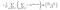Rearranged version

At the end of the algorithm, a measurement of the first register (quantum system) is made on a computational basis. To see what happens, consider the total amplitude (coefficient) of |z⟩=|0⟩⊗n in the first register of state |ψ3⟩. This amplitude is:

Consider this amplitude in the two cases: f constant and f balanced. If f is constant, the amplitude of |0⟩⊗n is either +1 or −1 (depending on what value f(x) takes). So if f is constant, a measurement of the first register is certain to return all 0s (by ‘all 0s’ we mean the binary string 00 ··· 0).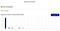Example of a Constant Measurement

I know that it is not 100% 0’s, but the problem in this situation is the ability to control the state of qubits at all times.

On the other hand, if f is balanced, then it is easy to see that the positive and negative contributions of the amplitudes cancel(through quantum interference), and the overall amplitude of |0⟩⊗n is 0. So if f is balanced, a measurement of the first register is certain not to return all 0s.

Even though have some fairly even values, which is what is expected if we get balanced as our result. There is still an error (so still not perfect).

As for the code, to determine whether f is constant or balanced, the first register is measured. If the result of the measurement is all 0s, then the algorithm outputs ‘constant’, and otherwise it outputs ‘balanced’.

16 yo TKS innovate wanting to give value to other people

## More from Ahmed Hassan

16 yo TKS innovate wanting to give value to other people